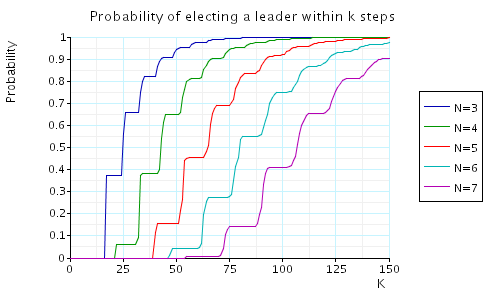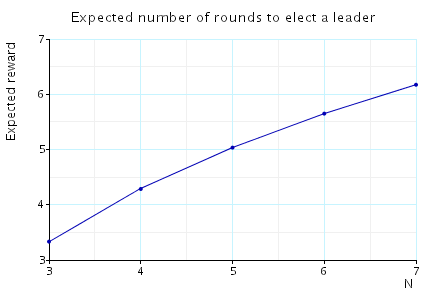(Itai & Rodeh)

### Introduction:

This case study is based on the asynchronous leader election protocol of Itai & Rodeh [IR90]. This protocol solves the following problem.

 Given an asynchronous ring of N processors design a protocol such that they will be able to elect a leader (a uniquely designated processor) by sending messages around the ring.

The protocol begins with all processors active. Each active process performs the following steps until it becomes inactive (when processors become inactive they only relay messages received):

 1 Choose 0 or 1 each with probability 0.5, and send the choice to the next processor. 2 If the processor chose 0 and the active processor preceding it in the ring chose 1 become inactive. 3 Send a counter around the ring to check whether it is the only active processor. If it is the only active processor then become leader, else repeat.

We assume that the ring is asynchronous: each processor has its own clock which has no relation to that of the other processors. Therefore, we cannot say that a message a processor wants to send will arrive at its destination processor at the same time slot. Instead, we assume that the order of the arrival of messages is governed by a scheduler which determines which message is sent next.

By way of example, the PRISM source code for the case where N=4 is given below.

```// asynchronous leader election
// 4 processes
// gxn/dxp 29/01/01

mdp

const N=4; // number of processes

module process1

// COUNTER
c1 : [0..N-1];

// STATES
s1 : [0..4];
// 0  make choice
// 1 have not received neighbours choice
// 2 active
// 3 inactive

// PREFERENCE
p1 : [0..1];

// VARIABLES FOR SENDING AND RECEIVING
sent1 : [0..2];
// not send anything
// sent choice
// sent counter

// pick value
[] (s1=0) -> 0.5 : (s1'=1) & (p1'=0) + 0.5 : (s1'=1) & (p1'=1);

// send preference
[p12] (s1=1) & (sent1=0) -> (sent1'=1);
// stay active
[p41] (s1=1) & (receive1=0) & !( (p1=0) & (p4=1) ) -> (s1'=2) & (receive1'=1);
// become inactive
[p41] (s1=1) & (receive1=0) & (p1=0) & (p4=1) -> (s1'=3) & (receive1'=1);

// send preference (can now reset preference)
[p12] (s1=2) & (sent1=0) -> (sent1'=1) & (p1'=0);
// send counter (already sent preference)
[c12] (s1=2) & (sent1=1) & (receive1=1) -> (sent1'=2);
[c12] (s1=2) & (sent1=1) & (receive1=2) -> (s1'=0) & (p1'=0) & (c1'=0) & (sent1'=0) & (receive1'=0);

// receive counter and not sent yet (note in this case do not pass it on as will send own counter)
// receive counter and sent counter
// only active process (decide)
[c41] (s1=2) & (receive1=1) & (sent1=2) & (c4=N-1) -> (s1'=4) & (p1'=0) & (c1'=0) & (sent1'=0) & (receive1'=0);
// other active process (pick again)
[c41] (s1=2) & (receive1=1) & (sent1=2) & (c4<N-1) -> (s1'=0) & (p1'=0) & (c1'=0) & (sent1'=0) & (receive1'=0);

// send preference (must have received preference) and can now reset
[p12] (s1=3) & (receive1>0) & (sent1=0) -> (sent1'=1) & (p1'=0);
// send counter (must have received counter first) and can now reset
[c12] (s1=3) & (receive1=2) & (sent1=1) ->  (s1'=3) & (p1'=0) & (c1'=0) & (sent1'=0) & (receive1'=0);

// done
[done] (s1=4) -> (s1'=s1);
// add loop for processes who are inactive
[done] (s1=3) -> (s1'=s1);

endmodule

// reward - expected number of rounds (equals the number of times a process receives a counter)
rewards "rounds"
[c12] true : 1;
endrewards
```

### Model Statistics

The table below shows statistics for the MDPs we have built for different values of the constants N and K. The tables include:

• the number of states and transitions in the MDP representing the model;
• both the number of nodes and leaves of the MTBDD which represents the model;
• the construction time which equals the time taken for the system description to be parsed and converted to the MTBDD representing it, and the time to perform reachability, identify the non-reachable states and filter the MTBDD accordingly;
• the number of iterations required to find the reachable states (which is performed via a BDD based fixpoint algorithm).
 N: Model: MTBDD: Construction: States: Transitions: Nodes: Leaves: Time (s): Iter.s: 3 364 654 3,345 3 0.146 18 4 3,172 7,144 10,747 3 0.341 31 5 27,299 74,365 31,414 3 1.407 42 6 237,656 760,878 77,980 3 5.664 61 7 2,095,783 7,714,385 180,361 3 20.91 77 8 18,674,484 77,708,080 392,068 3 79.37 94

### Model Checking:

In this case study we use the BDD based fixpoint algorithmProb0E, which find those states for which the maximum probability of satisfying the formula is greater than 0. When model checking, we have the following requirement:

• Scheduler-Liveness: for each time t and any processor i, there exists a time t' > t in which i is scheduled.

This corresponds to the conditions on the adversary/scheduler which we impose by model checking against only fair adversaries.

We have model checked the following properties:

• Only one leader is elected.

This is an invariance property and in the case of 4 processes corresponds to the PCTL formula:

(s1=4?1:0) + (s2=4?1:0) + (s3=4?1:0) + (s4=4?1:0)<=1

Model checking times:

 N: Time (s): 3 0.009 4 0.010 5 0.010 6 0.021 7 0.039 8 0.148

Conclusion: the property holds in all states
• With probability 1, eventually a leader is elected.

In the case of 4 processes this property can be expressed in PCTL as follows:

P>=1 [ true U (s1=4 | s2=4 | s3=4 | s4=4) ]

Model checking times:

 N: Prob0E precomputation: Time (s): Iterations: 3 0.076 24 4 0.53 33 5 3.02 51 6 13.8 62 7 55.5 76 8 198 90

Conclusion: the property holds in all states
• The minimum/maximum probability of electing a leader within k steps.

For the case of 4 processes these property can be expressed in PCTL as follows:

• Pmin=?[ true U<=K (s1=4 | s2=4 | s3=4 | s4=4) ]
• Pmax=?[ true U<=K (s1=4 | s2=4 | s3=4 | s4=4) ]

In the graph below we have plotted these probabilities as the number of processes varies. Note that the minimum and maximum probabilities are the same in each case.• The minimum/maximum expected number of rounds to elect a leader.

For the case of 4 processes these property can be expressed in PCTL as follows:

• Rmin=?[ F (s1=4 | s2=4 | s3=4 | s4=4) ]
• Rmax=?[ F (s1=4 | s2=4 | s3=4 | s4=4) ]

In the graph below we have plotted these expected values as the number of processes varies. Note that the minimum and maximum expected time are the same in each case.